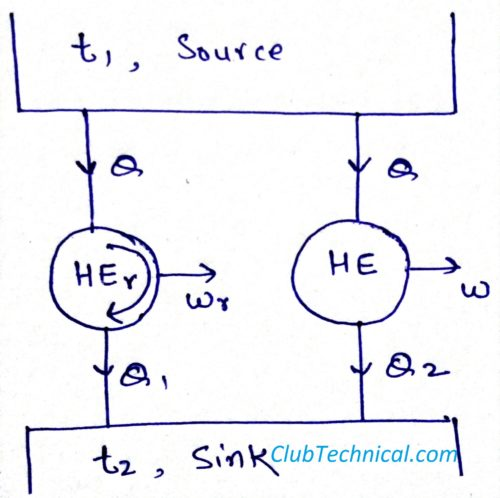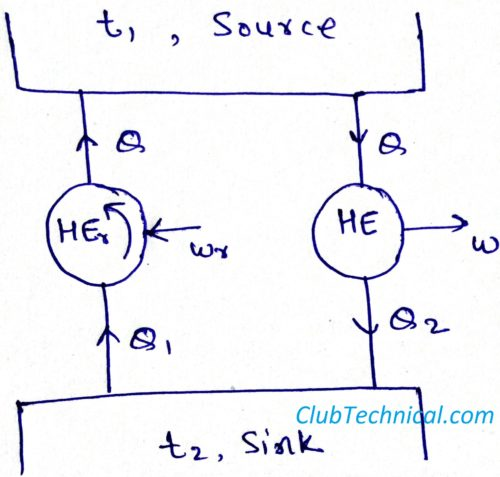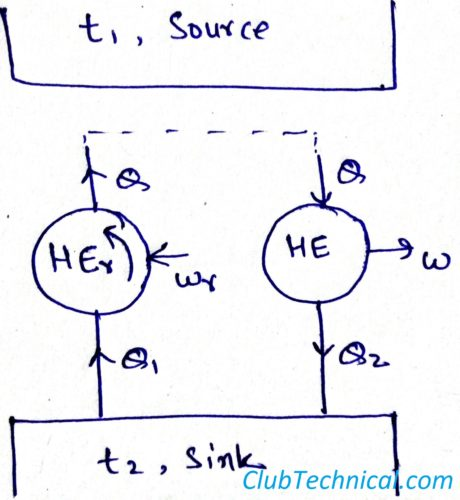# Carnot’s Theorem and its Proof [with Diagrams]

By | February 27, 2019

## Carnot theorem

No heat engine can be more efficient than a reversible engine operating between the same temperature limits (the temperature of heat addition and the temperature of heat rejection) and all reversible heat engines operating between the same temperature limits have the same efficiency.

## Proof of Carnot theorem

Given:

t1: Temperature of the source

t2: Temperature of the sink

Q: Heat supplied to the heat engine

Q1: Heat rejected by the reversible heat engine

Q2: Heat rejected by non-reversible heat engine

Wr: Work output of the reversible heat engine

W: Work output of the non-reversible heat engine

t1 > t2

Assumption:

Efficiency of non-reversible heat engine is more than efficiency of reversible heat engine.

Step 1:In the above diagram we can see there are two heat engines operating between the temperature limits t1 (source) and t2 (sink). They both are taking the same amount of heat input and generating some work.

According to our assumption work output by the non-reversible heat engine (W) is more than the work output of the reversible heat engine (Wr)

W > Wr

Step 2:We now reverse the reversible heat engine. Now it will become a heat pump and will take heat Wr from the surrounding and supply heat Q1 from sink to source.

Q = Q1 + Wr

Step 3:Since heat output of the reversible heat engine (now heat pump) and heat input of the non-reversible heat engine are the same, it means we can simply connect then bypassing the source.

Now the source becomes redundant.

We can see that the system is generating work(Wextra) by working on single fixed temperature. Which is a clear violation of second law of thermodynamics.

Wextra = W – Wr

It means that our assumption was wrong and efficiency of a reversible heat engine is always more than a non-reversible heat engine.

What is first law of thermodynamics?

What is a thermodynamic process?

What is energy (with respect to thermodynamics)?

What is thermodynamic equilibrium?

What is Gibbs’ phase rule?

What is a thermodynamic property?

This site uses Akismet to reduce spam. Learn how your comment data is processed.